StatLect

# Multinomial coefficient

The multinomial coefficient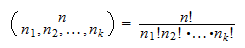is used to denote the number of possible partitions of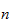objects into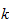groups having numerosity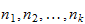.

The coefficient takes its name from the following multinomial expansion: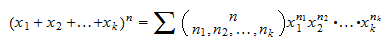whereand the sum is over all the-tuplessuch that: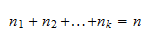## Example

The number of possible ways to subdivide six objects into three groups of two objects each is given by the multinomial coefficient## More details

The lecture entitled Partitions into groups provides an in-depth presentation of the multinomial coefficient.

Previous entry: Mean squared error

Next entry: Normal equations